Open in App
Not now

# Advantages of circular queue over linear queue

• Difficulty Level : Medium
• Last Updated : 18 Mar, 2021

Linear Queue: A Linear Queue is generally referred to as Queue. It is a linear data structure that follows the FIFO (First In First Out) order. A real-life example of a queue is any queue of customers waiting to buy a product from a shop where the customer that came first is served first. In Queue all deletions (dequeue) are made at the front and all insertions (enqueue) are made at the rear end.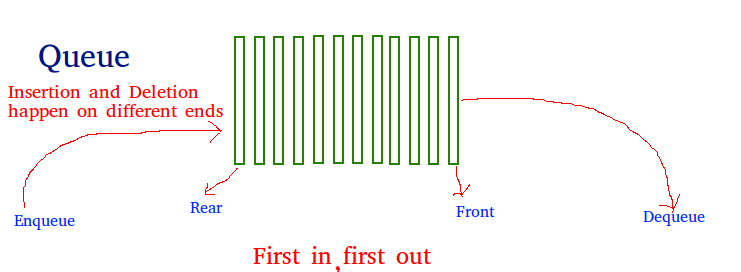Circular Queue: Circular Queue is just a variation of the linear queue in which front and rear-end are connected to each other to optimize the space wastage of the Linear queue and make it efficient.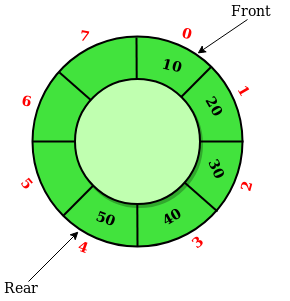Below are the operations that will illustrate that how is Circular Queue is better than Linear Queue:

• When Enqueue operation is performed on both the queues: Let the queue is of size 6 having elements {29, 21, 72, 13, 34, 24}. In both the queues the front points at the first element 29 and the rear points at the last element 24 as illustrated below: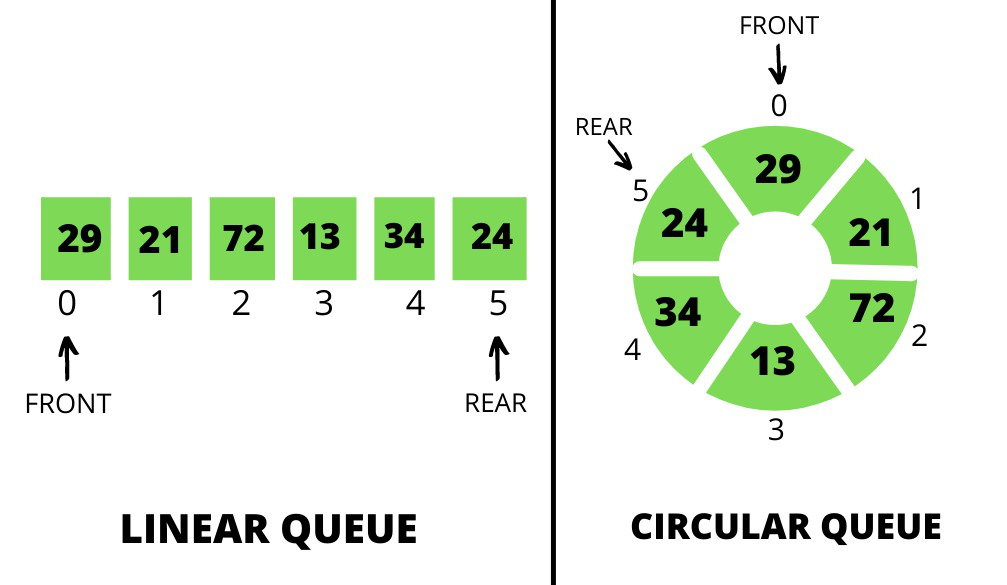• When the Dequeue operation is performed on both the queues: Consider the first 2 elements that are deleted from both the queues. In both the queues the front points at element 72 and the rear points at element 24 as illustrated below: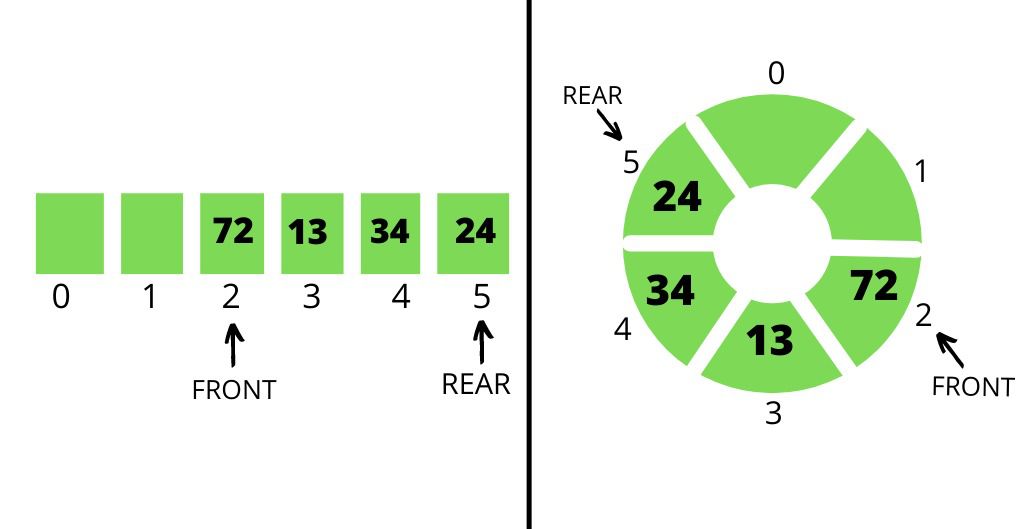• Now again enqueue operation is performed: Consider an element with a value of 100 is inserted in both the queues. The insertion of element 100 is not possible in Linear Queue but in the Circular Queue, the element with a value of 100 is possible as illustrated below: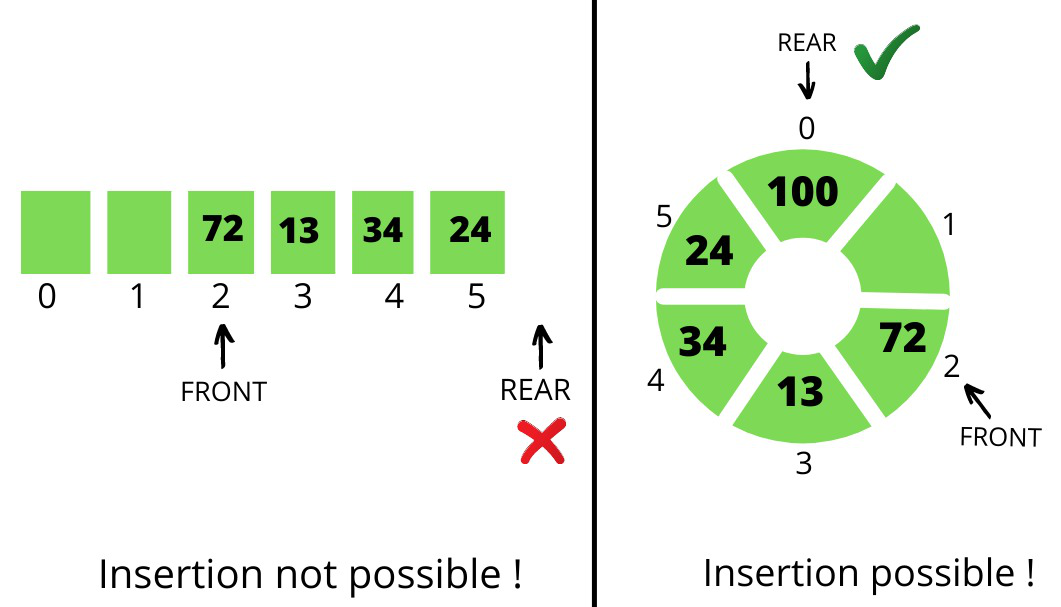• Explanation:

• As the insertion in the queue is from the rear end and in the case of Linear Queue of fixed size insertion is not possible when rear reaches the end of the queue.
• But in the case of Circular Queue, the rear end moves from the last position to the front position circularly.

Conclusion: The circular queue has more advantages than a linear queue. Other advantages of circular queue are:

• Easier for insertion-deletion: In the circular queue, elements can be inserted easily if there are vacant locations until it is not fully occupied, whereas in the case of a linear queue insertion is not possible once the rear reaches the last index even if there are empty locations present in the queue.
• Efficient utilization of memory: In the circular queue, there is no wastage of memory as it uses the unoccupied space, and memory is used properly in a valuable and effective manner as compared to a linear queue.
• Ease of performing operations: In the linear queue, FIFO is followed, so the element inserted first is the element to be deleted first. This is not the scenario in the case of the circular queue as the rear and front are not fixed so the order of insertion-deletion can be changed, which is very useful.
My Personal Notes arrow_drop_up